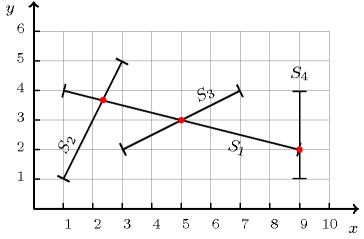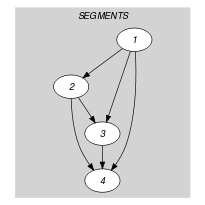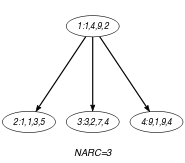## 5.95. crossing

Origin

Inspired by [CormenLeisersonRivest90].

Constraint

$\mathrm{𝚌𝚛𝚘𝚜𝚜𝚒𝚗𝚐}\left(\mathrm{𝙽𝙲𝚁𝙾𝚂𝚂},\mathrm{𝚂𝙴𝙶𝙼𝙴𝙽𝚃𝚂}\right)$

Arguments
 $\mathrm{𝙽𝙲𝚁𝙾𝚂𝚂}$ $\mathrm{𝚍𝚟𝚊𝚛}$ $\mathrm{𝚂𝙴𝙶𝙼𝙴𝙽𝚃𝚂}$ $\mathrm{𝚌𝚘𝚕𝚕𝚎𝚌𝚝𝚒𝚘𝚗}\left(\mathrm{𝚘𝚡}-\mathrm{𝚍𝚟𝚊𝚛},\mathrm{𝚘𝚢}-\mathrm{𝚍𝚟𝚊𝚛},\mathrm{𝚎𝚡}-\mathrm{𝚍𝚟𝚊𝚛},\mathrm{𝚎𝚢}-\mathrm{𝚍𝚟𝚊𝚛}\right)$
Restrictions
 $\mathrm{𝙽𝙲𝚁𝙾𝚂𝚂}\ge 0$ $\mathrm{𝙽𝙲𝚁𝙾𝚂𝚂}\le \left(|\mathrm{𝚂𝙴𝙶𝙼𝙴𝙽𝚃𝚂}|*|\mathrm{𝚂𝙴𝙶𝙼𝙴𝙽𝚃𝚂}|-|\mathrm{𝚂𝙴𝙶𝙼𝙴𝙽𝚃𝚂}|\right)/2$ $\mathrm{𝚛𝚎𝚚𝚞𝚒𝚛𝚎𝚍}$$\left(\mathrm{𝚂𝙴𝙶𝙼𝙴𝙽𝚃𝚂},\left[\mathrm{𝚘𝚡},\mathrm{𝚘𝚢},\mathrm{𝚎𝚡},\mathrm{𝚎𝚢}\right]\right)$
Purpose

$\mathrm{𝙽𝙲𝚁𝙾𝚂𝚂}$ is the number of line segments intersections between the line segments defined by the $\mathrm{𝚂𝙴𝙶𝙼𝙴𝙽𝚃𝚂}$ collection. Each line segment is defined by the coordinates $\left(\mathrm{𝚘𝚡},\mathrm{𝚘𝚢}\right)$ and $\left(\mathrm{𝚎𝚡},\mathrm{𝚎𝚢}\right)$ of its two extremities.

Example
$\left(\begin{array}{c}3,〈\begin{array}{cccc}\mathrm{𝚘𝚡}-1\hfill & \mathrm{𝚘𝚢}-4\hfill & \mathrm{𝚎𝚡}-9\hfill & \mathrm{𝚎𝚢}-2,\hfill \\ \mathrm{𝚘𝚡}-1\hfill & \mathrm{𝚘𝚢}-1\hfill & \mathrm{𝚎𝚡}-3\hfill & \mathrm{𝚎𝚢}-5,\hfill \\ \mathrm{𝚘𝚡}-3\hfill & \mathrm{𝚘𝚢}-2\hfill & \mathrm{𝚎𝚡}-7\hfill & \mathrm{𝚎𝚢}-4,\hfill \\ \mathrm{𝚘𝚡}-9\hfill & \mathrm{𝚘𝚢}-1\hfill & \mathrm{𝚎𝚡}-9\hfill & \mathrm{𝚎𝚢}-4\hfill \end{array}〉\hfill \end{array}\right)$

Figure 5.95.1 provides a picture of the example with the corresponding four line segments of the $\mathrm{𝚂𝙴𝙶𝙼𝙴𝙽𝚃𝚂}$ collection. The $\mathrm{𝚌𝚛𝚘𝚜𝚜𝚒𝚗𝚐}$ constraint holds since its first argument $\mathrm{𝙽𝙲𝚁𝙾𝚂𝚂}$ is set to 3, which is actually the number of line segments intersections.

##### Figure 5.95.1. Illustration of the Example slot: intersection, in red, between the four line segments ${S}_{1}$, ${S}_{2}$, ${S}_{3}$ and ${S}_{4}$ ($\mathrm{𝙽𝙲𝚁𝙾𝚂𝚂}=3$)Typical
$|\mathrm{𝚂𝙴𝙶𝙼𝙴𝙽𝚃𝚂}|>1$
Symmetries
• Items of $\mathrm{𝚂𝙴𝙶𝙼𝙴𝙽𝚃𝚂}$ are permutable.

• Attributes of $\mathrm{𝚂𝙴𝙶𝙼𝙴𝙽𝚃𝚂}$ are permutable w.r.t. permutation $\left(\mathrm{𝚘𝚡},\mathrm{𝚘𝚢}\right)$ $\left(\mathrm{𝚎𝚡},\mathrm{𝚎𝚢}\right)$ (permutation applied to all items).

• One and the same constant can be added to the $\mathrm{𝚘𝚡}$ and $\mathrm{𝚎𝚡}$ attributes of all items of $\mathrm{𝚂𝙴𝙶𝙼𝙴𝙽𝚃𝚂}$.

• One and the same constant can be added to the $\mathrm{𝚘𝚢}$ and $\mathrm{𝚎𝚢}$ attributes of all items of $\mathrm{𝚂𝙴𝙶𝙼𝙴𝙽𝚃𝚂}$.

Arg. properties

Functional dependency: $\mathrm{𝙽𝙲𝚁𝙾𝚂𝚂}$ determined by $\mathrm{𝚂𝙴𝙶𝙼𝙴𝙽𝚃𝚂}$.

Keywords
Arc input(s)

$\mathrm{𝚂𝙴𝙶𝙼𝙴𝙽𝚃𝚂}$

Arc generator
$\mathrm{𝐶𝐿𝐼𝑄𝑈𝐸}$$\left(<\right)↦\mathrm{𝚌𝚘𝚕𝚕𝚎𝚌𝚝𝚒𝚘𝚗}\left(𝚜\mathtt{1},𝚜\mathtt{2}\right)$

Arc arity
Arc constraint(s)
 $•\mathrm{𝚖𝚊𝚡}\left(𝚜\mathtt{1}.\mathrm{𝚘𝚡},𝚜\mathtt{1}.\mathrm{𝚎𝚡}\right)\ge \mathrm{𝚖𝚒𝚗}\left(𝚜\mathtt{2}.\mathrm{𝚘𝚡},𝚜\mathtt{2}.\mathrm{𝚎𝚡}\right)$ $•\mathrm{𝚖𝚊𝚡}\left(𝚜\mathtt{2}.\mathrm{𝚘𝚡},𝚜\mathtt{2}.\mathrm{𝚎𝚡}\right)\ge \mathrm{𝚖𝚒𝚗}\left(𝚜\mathtt{1}.\mathrm{𝚘𝚡},𝚜\mathtt{1}.\mathrm{𝚎𝚡}\right)$ $•\mathrm{𝚖𝚊𝚡}\left(𝚜\mathtt{1}.\mathrm{𝚘𝚢},𝚜\mathtt{1}.\mathrm{𝚎𝚢}\right)\ge \mathrm{𝚖𝚒𝚗}\left(𝚜\mathtt{2}.\mathrm{𝚘𝚢},𝚜\mathtt{2}.\mathrm{𝚎𝚢}\right)$ $•\mathrm{𝚖𝚊𝚡}\left(𝚜\mathtt{2}.\mathrm{𝚘𝚢},𝚜\mathtt{2}.\mathrm{𝚎𝚢}\right)\ge \mathrm{𝚖𝚒𝚗}\left(𝚜\mathtt{1}.\mathrm{𝚘𝚢},𝚜\mathtt{1}.\mathrm{𝚎𝚢}\right)$ $•\bigvee \left(\begin{array}{c}\begin{array}{c}\left(𝚜\mathtt{2}.\mathrm{𝚘𝚡}-𝚜\mathtt{1}.\mathrm{𝚎𝚡}\right)*\left(𝚜\mathtt{1}.\mathrm{𝚎𝚢}-𝚜\mathtt{1}.\mathrm{𝚘𝚢}\right)-\hfill \\ \left(𝚜\mathtt{1}.\mathrm{𝚎𝚡}-𝚜\mathtt{1}.\mathrm{𝚘𝚡}\right)*\left(𝚜\mathtt{2}.\mathrm{𝚘𝚢}-𝚜\mathtt{1}.\mathrm{𝚎𝚢}\right)\hfill \end{array}=0,\hfill \\ \begin{array}{c}\left(𝚜\mathtt{2}.\mathrm{𝚎𝚡}-𝚜\mathtt{1}.\mathrm{𝚎𝚡}\right)*\left(𝚜\mathtt{2}.\mathrm{𝚘𝚢}-𝚜\mathtt{1}.\mathrm{𝚘𝚢}\right)-\hfill \\ \left(𝚜\mathtt{2}.\mathrm{𝚘𝚡}-𝚜\mathtt{1}.\mathrm{𝚘𝚡}\right)*\left(𝚜\mathtt{2}.\mathrm{𝚎𝚢}-𝚜\mathtt{1}.\mathrm{𝚎𝚢}\right)\hfill \end{array}=0,\hfill \\ \begin{array}{c}\mathrm{𝚜𝚒𝚐𝚗}\left(\begin{array}{c}\begin{array}{c}\left(𝚜\mathtt{2}.\mathrm{𝚘𝚡}-𝚜\mathtt{1}.\mathrm{𝚎𝚡}\right)*\left(𝚜\mathtt{1}.\mathrm{𝚎𝚢}-𝚜\mathtt{1}.\mathrm{𝚘𝚢}\right)-\hfill \\ \left(𝚜\mathtt{1}.\mathrm{𝚎𝚡}-𝚜\mathtt{1}.\mathrm{𝚘𝚡}\right)*\left(𝚜\mathtt{2}.\mathrm{𝚘𝚢}-𝚜\mathtt{1}.\mathrm{𝚎𝚢}\right)\hfill \end{array}\hfill \end{array}\right)\ne \hfill \\ \mathrm{𝚜𝚒𝚐𝚗}\left(\begin{array}{c}\begin{array}{c}\left(𝚜\mathtt{2}.\mathrm{𝚎𝚡}-𝚜\mathtt{1}.\mathrm{𝚎𝚡}\right)*\left(𝚜\mathtt{2}.\mathrm{𝚘𝚢}-𝚜\mathtt{1}.\mathrm{𝚘𝚢}\right)-\hfill \\ \left(𝚜\mathtt{2}.\mathrm{𝚘𝚡}-𝚜\mathtt{1}.\mathrm{𝚘𝚡}\right)*\left(𝚜\mathtt{2}.\mathrm{𝚎𝚢}-𝚜\mathtt{1}.\mathrm{𝚎𝚢}\right)\hfill \end{array}\hfill \end{array}\right)\hfill \end{array}\hfill \end{array}\right)$
Graph property(ies)
$\mathrm{𝐍𝐀𝐑𝐂}$$=\mathrm{𝙽𝙲𝚁𝙾𝚂𝚂}$

Graph class
 $•$$\mathrm{𝙰𝙲𝚈𝙲𝙻𝙸𝙲}$ $•$$\mathrm{𝙽𝙾}_\mathrm{𝙻𝙾𝙾𝙿}$

Graph model

Each line segment is described by the $𝚡$ and $𝚢$ coordinates of its two extremities. In the arc generator we use the restriction $<$ in order to generate a single arc for each pair of segments. This is required, since otherwise we would count more than once a given line segments intersection.

Parts (A) and (B) of Figure 5.95.2 respectively show the initial and final graph associated with the Example slot. Since we use the $\mathrm{𝐍𝐀𝐑𝐂}$ graph property, the arcs of the final graph are stressed in bold. An arc constraint expresses the fact the two line segments intersect. It is taken from [CormenLeisersonRivest90]. Each arc of the final graph corresponds to a line segments intersection.

##### Figure 5.95.2. Initial and final graph of the $\mathrm{𝚌𝚛𝚘𝚜𝚜𝚒𝚗𝚐}$ constraint(a) (b)